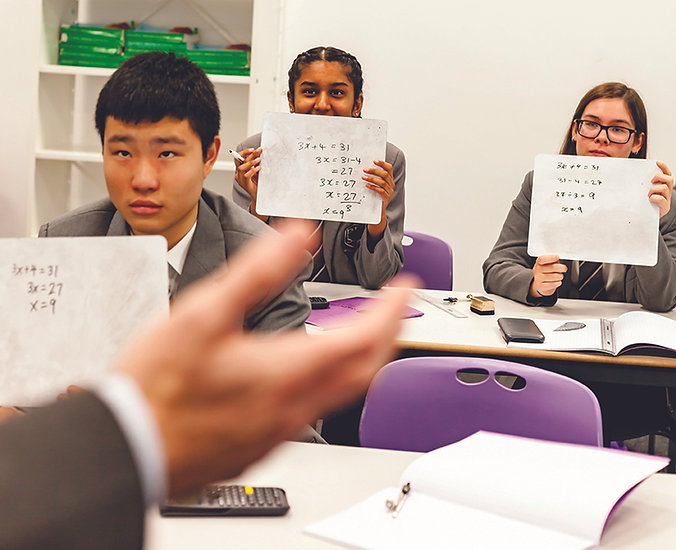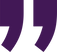# Mathematics

Mathematics is a creative and highly interconnected discipline that has been developed over centuries, providing the solution to some of history’s most intriguing problems.

It is essential to everyday life, critical to science, technology and engineering, and necessary for financial literacy and most forms of employment.

# Back to Subjects## beauty.Bertrand Russell

We provide a sequenced mathematics curriculum that inspires curiosity, allows for collaboration and develops student’s resilience whilst developing their understanding. Our curriculum develops fluent knowledge, skills and understanding of mathematical methods and concepts. It allows students to acquire, select and apply mathematical techniques to solve problems. Gives students the opportunity to reason mathematically, make deductions and inferences, and draw conclusions.

## Students at RFSS will be able to:

• The ability to think critically about mathematics and communicate confidently through their writing and oracy.

• The ability to support, evaluate and challenge their own and others’ views using detailed mathematical proof and evidence derived from a range of contexts.

## What are they learning?

### Year 7

Students will build on their primary mathematical education by considering deeper, relational aspects of arithmetic before beginning to generalise those algebraically and apply to a variety of contexts (for example, perimeter and area as well as financial statements). We follow the White Rose Maths Scheme of Learning, and students will study the following 'blocks':

• Sequences
• Understand and use algebraic notation
• Equality and equivalence
• Place value and ordering integers and decimals
• Fraction, decimal and percentage equivalence
• Solving problems with addition & subtraction
• Solving problems with multiplication & division
• Fractions and percentages of amounts
• Operations and equations with directed numbers
• Addition and subtraction of fractions
• Constructing, measuring and using geometric notation
• Developing geometric reasoning
• Developing number sense
• Sets and Probability
• Prime numbers and proof

### Year 9

Currently, students begin their GCSE course in year 9. The GCSE course builds on understanding started in KS3 to further develop existing strands of mathematics (namely Number, Ratio, Algebra, Geometry and Measure and Statistics) in both abstract and practical directions.

### Potential Careers relating to your subject

Engineering / Accountancy / Investment Banking / Business Leadership / Economist / Scientist / Electrician / Carpentry / Data Analyst / Computer Programmer

### Year 8

Students build on their understanding of arithmetic and algebra and also begin to incorporate understanding of ratio and graphs into their canon of knowledge. The blocks in year 8 are:

• Ratio and scale
• Multiplicative change
• Multiplying and dividing fractions
• Working in the Cartesian plane
• Representing data
• Tables and probability
• Brackets, equations and inequalities
• Sequences
• Indices
• Fractions and percentages
• Standard Index Form
• Number sense
• Angles in parallel lines and polygons
• Area of trapezia and circles
• Line symmetry and reflection
• The data handling cycle
• Measures of location

### GCSE

A vast amount of content is covered at GCSE at either foundation or higher tier. The content is broadly structured into the following six groupings: 1. Number 2. Algebra 3. Ratio, proportion and rates of change 4. Geometry and Measure 5. Probability 6. Statistics. Some of the most famous results in mathematics are built up to and introduced to students at both tiers - for example, Pythagoras' Theorem - and extended in the higher tier - for example, extending Pythagoras' Theorem and building the Cosine Rule. Additionally, students gain a set of practical mathematical skills such as interpreting financial statements and analysing and interpreting statistical output. The full detailed specification can be found on the exam board's website at: https://qualifications.pearson.com/content/dam/pdf/GCSE/mathematics/2015/specification-and-sample-assesment/gcse-maths-2015-specification.pdf